# Excel DSUMArticle byExcelMojo Team## What Is DSUM Function In Excel?

The Excel DSUM Function evaluates the sum of a field or column of data based on a specified criteria. The function is an alternative to the SUMIF and the SUMIFS functions in Excel.

The DSUM Function is categorized under the Excel Database functions of the Function library. The DSUM function was introduced in MS Excel 2000. For example, suppose we have a database of products and their sales. We will calculate the database Sum using the DSUM Excel Function.

The DSUM formula =DSUM(A1:C4,“Sales”,E1:F2) is entered in cell G2. The result is returned as ‘100.’

###### Key Takeaways
• The DSUM Function in Excel is an alternative formula for the SUMIF and SUMIFS functions.
• If the specified criteria are not set, the function generates the sum of the overall column.
• The drop-down list is created according to the criteria to get different regions or rep totals.
• As the drop-down list changes, it may show the results accordingly.
• The criteria table in the data is not case-sensitive.

### DSUM() Excel Formula

The syntax of the Excel DSUM Formula  is

• database: This is a mandatory argument and indicates the range of cells that specifies the first row of the data showing the field names.
• field: This is a mandatory argument and shows the column we want to summarize in the table.
• criteria: This is a mandatory argument and is the criteria in the cells containing the conditions.

### How To Use DSUM Excel Function?

#### 1. Access From The Excel Ribbon

• Choose the empty cell which will contain the result.
• Go to the Formulas tab and click it.
• Select the Database option.
• Select DSUM from the menu.
• A window called Function Arguments appears.
• As the number of arguments, enter the value in the “database,” “field,” & “criteria.”
• Select OK.

#### 2. Enter The Worksheet Manually

1. Select an empty cell for the output.
2. Type =DSUM( in the selected cell. Alternatively, type =D and double-click the DSUM function from the list of suggestions shown by Excel.
3. Press Enter key.

Let us take an example to understand this function.

The succeeding example depicts some fruits and their quantity. We will find the database Sum using the DSUM Function.

In the table,

• Column A shows the fruits.
• Column B highlights the quantity.
• Column E contains the output.

The steps to evaluate the values by the Excel DSUM Function are as follows:

1. Select the cell where we will enter the formula and calculate the result. The selected cell, in this case, is cell E2.

2. Next, we will enter the Excel DSUM Formula in cell E2.

3. Enter the value of ‘database’ as A1:B6, i.e., the needed database value.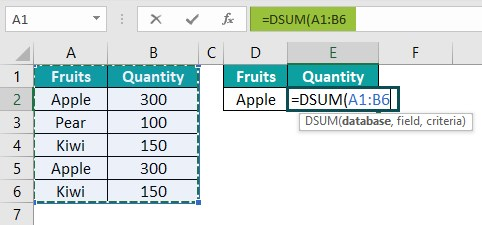4. Enter the value of the ‘field’ as “Quantity,” i.e., the quantity we want to perform the calculation.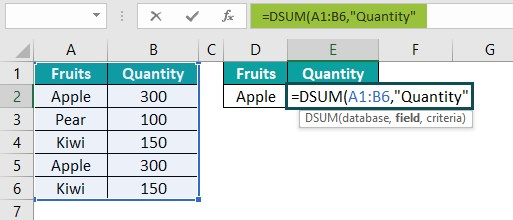5. Enter the value of the ‘criteria’ as “D1:D2,” i.e., the checked value we want to calculate.

6. The complete formula is =DSUM(A1:B6,”Quantity”,D1:D2) in cell E2.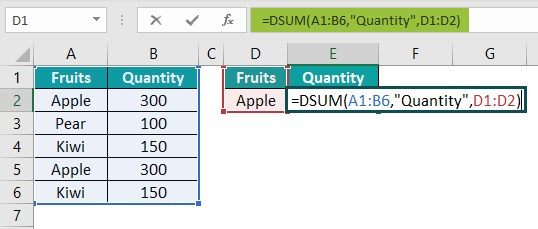7. After entering each value in the preceding step, press Enter key. The results in cell E2 are “600” in the image below.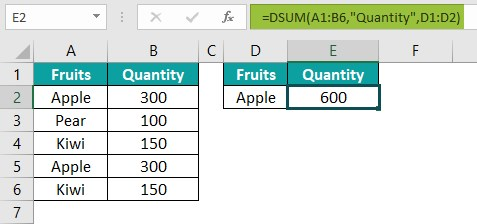### Examples

#### Example #1

The succeeding example depicts the items and total cost, and we will find the database sum using the Excel DSUM Function.

In the table, the data is reflected below: –

• Column A contains the Region.
• Column B contains the Item.
• Column C contains the Unit.
• Column D contains the Total Cost.
• Column G contains the Output.

The steps to evaluate the values by the DSUM Function are as follows:

Step 1: Select the cell where we will enter the formula and calculate the result. The selected cell, in this case, is cell G2.

Step 2:  Next, we will enter the Excel DSUM Formula in cell G2.

Step 3: Enter the value of ‘database’ as A1:D5, i.e., the needed database value.

Step 4: Enter the value of the ‘field’ as “Total Cost,” i.e., the quantity we want to perform the calculation.

Step 5: Enter the value of the ‘criteria’ as “F1:F2,” i.e., the checked value we want to calculate.

Step 6: The complete formula is =DSUM(A1:D5,”Total Cost”,F1:F2) in cell G2.

Step 7: After entering each value in the preceding step, press the Enter key. The results in cell G2 are \$2,00,20,693 in the image below.

#### Example#2

The succeeding example depicts the movies and the Oscars prize winners. Let us use the DSUM method to find the sum of the Oscars won by a specific film.

In the table, the data is reflected below: –

• Column A contains the Movie.
• Column B contains the Year.
• Column C contains the Oscar Won.
• Column G contains the Output.

The steps to evaluate the values by the Excel DSUM Function are as follows:

Step 1: Select the cell where we will enter the formula and calculate the result. The selected cell, in this case, is cell G2.

Step 2:  Next, we will enter the Excel DSUM Formula in cell G2.

Step 3: Enter the value of ‘database’ as A1:C6, i.e., the needed database value.

Step 4: Enter the value of the ‘field’ as “Oscars Won,” i.e., the won prizes in which we want to perform the calculation.

Step 5: Enter the value of the ‘criteria’ as “F1:F2,” i.e., the checked value we want to calculate.

Step 6: The complete formula is =DSUM(A1:C6,”Oscars Won”,F1:F2) in cell G2.

Step 7: After entering each value in the preceding step, press Enter key. The results in cell G2 are “12” in the image below.

#### Example#3

The succeeding example depicts the departmental sales of the year, and we will find the database sum using the DSUM Function.

In the table, the data is reflected below: –

• Column A contains the Department.
• Column B contains the Year.
• Column C contains the Sales.
• Column F contains the Output.

The steps to evaluate the values by the Excel DSUM Function are as follows:

Step 1: Select the cell where we will enter the formula and calculate the result. The selected cell, in this case, is cell F2.

Step 2:  Next, we will enter the Excel DSUM Formula in cell F2.

Step 3: Enter the value of ‘database’ as A1:C5, i.e., the needed database value.

Step 4: Enter the value of the ‘field’ as “Sales,” i.e., the sales in which we want to perform the calculation.

Step 5: Enter the value of the ‘criteria’ as “E1:E2,” i.e., the checked value we want to calculate.

Step 6: The complete formula is =DSUM(A1:C5,”Sales”,E1:E2) in cell F2.

Step 7: After entering each value in the preceding step, press Enter key. The results in cell F2 are “\$1,08,42,313” in the image below.

### Important Things To Note

• The criteria range should not be placed below the list. If we add information to the list, it is added to the first row below the list. Excel cannot add new information if the row below the list is blank.
• The criteria range should not overlap the list.
• If we want to operate the entire column in the given data, we must enter a blank line below the column labels in the criteria range.
• Firstly, identify the criteria requirements and list all the criteria.
• The table is created if the data size increases; it will be a dynamic range.

1. What does the DSUM function do in Excel?

The DSUM Function in Excel is also known as the DATABASE SUM function in Excel. The function calculates the given database’s sum based on a certain field and provided criteria. This function takes three arguments as inputs: the range for the database, an argument for the field, and a condition, and then it calculates the sum for it.

To calculate the total number of entered records, if the order number is greater than 100 and the quantity is greater than or equal to 10, the DSUM Excel formula calculates the sum of such orders.

The syntax of the DSUM function is as follows;
=DSUM(database,field,criteria)

2. How does the DSUM function work in Excel?

The DSUM function works in Excel by following the below steps;

1. Select an empty cell for the output.
2. Type =DSUM( in the selected cell. Alternatively, type =D and double-click the DSUM function from the list of suggestions shown by Excel.
3. Press Enter key.

For example, suppose we have a database of vegies and their sales. We will calculate the Database Sum using Excel DSUM Function.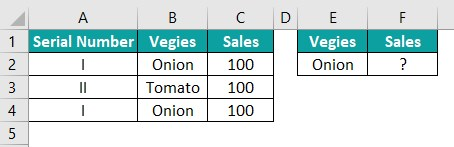The DSUM formula =DSUM(A1:C4,“Sales”,E1:E2) is entered in cell F2;

The result is returned as ‘200.’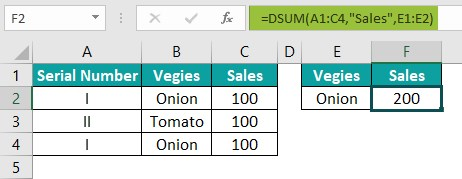3. Where is the DSUM Function in Excel?

We can insert Excel DSUM Function using the following steps:

• Choose the empty cell which will contain the result.
• Go to the Formulas tab and click it.
• Select the Database option from the drop-down menu.
• Select DSUM from the menu.
• A window called Function Arguments appears.
• As the number of arguments, enter the value in the “database,” “field,” & “criteria.”
• Select OK.